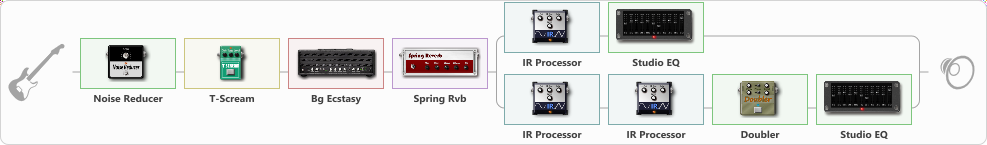# Bogner bald tone

Discussion in 'ToneLib-GFX presets' started by Metalhe4d, Oct 30, 2022.

1. Bogner bald tone

Preset name: Bogner Ecstasy

Effects chain:Effect: "Noise Reducer" (Dynamics / Filter), active - "yes"
{
"Sens" = 100
"Mode" = Soft
}

Effect: "T-Scream" (Overdrive / Distortion), active - "yes"
{
"Drive" = 74
"Tone" = 79
"Level" = 79
}

Effect: "Bg Ecstasy" (Amp simulators), active - "yes"
{
"Gain" = 76
"Bass" = 72
"Middle" = 24
"Treble" = 84
"Presence" = 100
"Master" = 74
"Level (dB)" = 0
}

Effect: "Spring Rvb" (Reverberation), active - "yes"
{
"Time" = 5.3
"PreDelay" = 0
"LoDamp" = 16
"HiDamp" = 26
"Mix" = 32
}

Effect: "Splitter" (Dynamics / Filter)
{
"A-Bypass" = Off
"A-Pan" = -30
"A-Level" = 55
"B-Bypass" = Off
"B-Pan" = 30
"B-Level" = 64
"Width" = 0

'A' branch:
{

Effect: "IR Processor" (Cabinets), active - "yes"
{
"IR" = Diezel V30 SM57 (6505 PowerAmp)
"Low Cut (Hz)" = 0
"Hi Cut (kHz)" = 12.5
"Mix" = 100
"Level (dB)" = 0
}

Effect: "Studio EQ" (Dynamics / Filter), active - "yes"
{
"31 Hz" = 0
"62 Hz" = 2
"125 Hz" = 0
"250 Hz" = 0
"500 Hz" = 0
"1 kHz" = 0
"2 kHz" = 0
"4 kHz" = 0
"8 kHz" = -4
"16 kHz" = 0
"Level (dB)" = 0
}
}
'B' branch:
{

Effect: "IR Processor" (Cabinets), active - "yes"
{
"IR" = Yamaha_LL16_48000_Match
"Low Cut (Hz)" = 0
"Hi Cut (kHz)" = 20.0
"Mix" = 100
"Level (dB)" = 0
}

Effect: "IR Processor" (Cabinets), active - "yes"
{
"IR" = Rev IR Sample5
"Low Cut (Hz)" = 0
"Hi Cut (kHz)" = 15.0
"Mix" = 45
"Level (dB)" = 0
}

Effect: "Doubler" (Dynamics / Filter), active - "yes"
{
"A-Dly" = 70
"A-Inv" = Off
"A-Level" = 50
"B-Dly" = 50
"B-Inv" = Off
"B-Level" = 50
}

Effect: "Studio EQ" (Dynamics / Filter), active - "yes"
{
"31 Hz" = 0
"62 Hz" = 6
"125 Hz" = 0
"250 Hz" = 0
"500 Hz" = 0
"1 kHz" = 0
"2 kHz" = 0
"4 kHz" = 0
"8 kHz" = 3
"16 kHz" = 0
"Level (dB)" = 0
}
}
}

Note: You will need to download and install the ToneLib-GFX software to use the preset.

File size:
149.2 KB
Views:
2,782